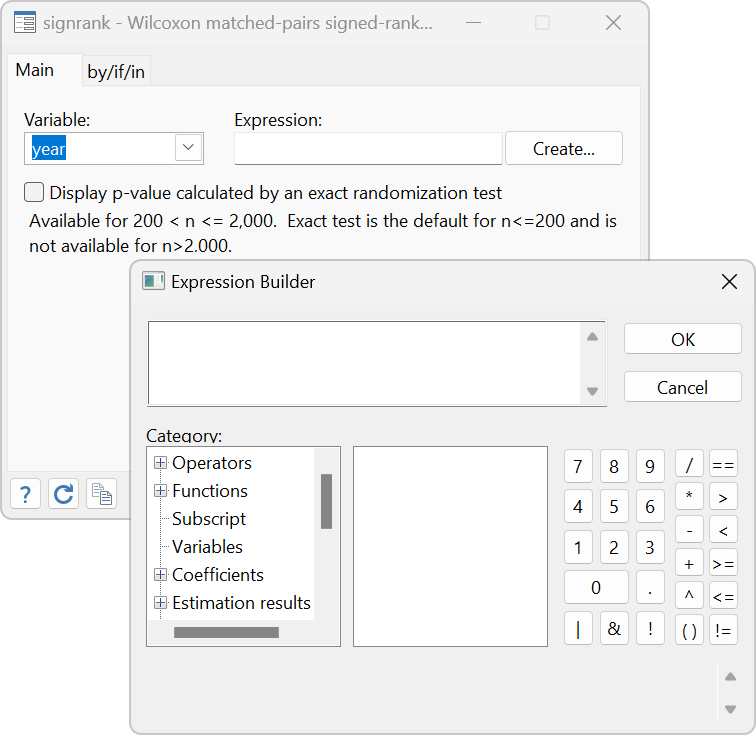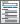»  Home »  Products »  Features »  Nonparametric methods

## Nonparametric methods

Nonparametric testsCentiles and confidence intervals• Estimate percentiles
• Exact confidence intervals
• Optionally assume normality

Resampling and simulations

Smoothing

• Multiple covariates supported
• Continuous covariates (8 kernels available)
• Discrete covariates (2 kernels available)
• Techniques
• local constant regression
• local linear regression
• Point estimates with SEs and CIs
• Derivative estimates with SEs and CIs
• Automatic optimal bandwidth selection
• Interface to margins for advanced inference
• Estimates of population-averaged and subpopulation-averaged means and effects
• Fully conditional means and effects at
• any specified values of the covariates
• Confidence intervals
• Tests
• Graphs via marginsplot
• Multiple covariates supported
• Continuous covariates
• Discrete covariates
• Series approximation with series
• B-splines basis
• Natural splines
• Polynomials
• Semiparametric regression models
• Optimal knot and polynomial selection
• cross-validation
• generalized cross-validation
• AIC
• BIC
• Mallows's Cp
• Interface to margins
• Estimates of population and subpopulation means and effects
• Fully conditional means and effects
• Confidence intervals
• Graphs via marginsplot

Nonparametric correlation coefficients

Survival analysis

Multivariate analysis

Treatment effects